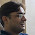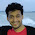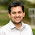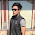## Feb 25, 2012

### Maxima Property Subset

Source: Asked to me by Santosh Ananthakrishnan (EE IITB Fifth year undergraduate, To be Worldquant Analyst)

Problem:
At most, how many subsets can you find of the set A = {1, 2, ..., n} such that any two intersect in exactly one element?

1.is it 2 raised to n-1??
because u can include one element in all the sets and then u have n-1 elements left , which can have 2^n-1 subsets....

2.Is the set A itself is excluded from the set of those subsets? Because the answer will be different in that case.

3.For each set in the family, let v be a n-length binary vector that indicates which elements of {1,2,...,n} belong to that set. Then, the intersection criterion becomes v_i^T v_j = 1. Let K be the largest collection of sets satisfying this criterion. If we can find the rank of {v_1,v_2,...,v_K} as a function of K, this will have to be upper bounded by n and we will have an upper bound on K.

Some experimentation shows that K=n is possible. Take the family to be {{1,2,3,...,n-1},{1,n},{2,n},...{n-1,n}}. For n=2, it is easy to see that we can't do better than K=2. This suggests that we should try to prove that the vectors v1,v2...,v_K are linearly independent.

Computing the Gram determinant of these basis vectors is easy. The diagonal elements are the cardinalities of the sets in the family and all off-diagonals are 1. The determinant of this matrix is P + \sum_{i=1}^n P/(|A_i|-1) where P = \prod_{i=1}^n (|A_i|-1). Thus, as long as the sets are not singletons, the vectors will be linearly independent. QED.

Coming up with a non-easy example (other than the example above and {{1,n},{2,n}...{n-1,n},{n}}) of such families is an interesting exercise in its own right.

4.A similar approach:
The characteristic functions of the sets can be viewed as vectors in n dimensions (with the added restriction that all components are either 1 or 0).

Upper bound:
Consider solving the problem: Given an n-dimensional space, find the largest set S of vectors such that the pairwise dot product is a.

We attempt a problem reduction as follows: Choose an arbitrary vector from the set, v. Rotate the space such that v points along one of the axes, say x. This does not change the pairwise dot product. Let the length of v be b. Now, since the dot product of v with all other vectors is a, for any other vector w in S, w . x = a / b.
Therefore, we must now solve the problem - find the set S' of vectors in n - 1 dimensions such that the pairwise dot product is a - a/ b.

This problem makes sense when a > a / b (This is always true). In the best case, we can assume that the dot product does not reduce in each step. Since each step reduces the number of dimensions by 1, |S| is upper bounded by n.

Lower bound: Example - The vectors
1 0 0 0 ...
1 1 0 0 0 ...
1 0 1 0 0 ...
1 0 0 1 0 ...
i.e.
x_0 = 1, x_i (i > 0) = 0;
x_0 = 1, x_i (unique i) = 1;

satisfy the conditions. This set is of size n.

5.2^n-2 if sum of elements of subset should be n
otherwise

n-2
Σ r(n-r-1)
r=1

6.Any 3 element subset, say (i,j,k) cannot be in the answer, because you can remove it and include (i,j) and (j,k) instead. Same holds for subsets with 4,5,... elements.

7."any 2 should intersect in exactly 1 element".

I should change my answer to C(n,2).

8.n*3^(n-1)
Find number of subsets such that element 1 is common between them. It is equivalent to choosing 2 disjoint subsets from remaining n-1 elements which is 3^(n-1).
Do that for element in the set.

9.i think it is n*2^(n-1) using the same logic as above.

10.n

explanation:
1 element can be chosen to shared.
then there be a maximum of n-1 disjoint sets of the left out n-1 elements, now if removed element is added to each of these sets we have n-1 subsets, the removed element itself will intersect with the rest of subsets in only one element,
hence the total no of subsets is n.

Incresing the size of any of these sets decreases the number of disjoint sets available, so the number of elements in each set should be kept to bare minumum ie 1

11.i think it's n-1.
n-1 different elements in n-1 different subsets while remaining 1 element with each of the n-1 subsets.

12.The answer should be n.I agree with Karthi's logic and explanation.
@gj: You forgot the n-th element could individually forma a subset as well

13.It should be n, the only way this seems possible is if (n-1) elements are taken in one of the subsets, and in each of the remaining subsets, the one element left out in the first subset is paired with the remaining elements of the first subset. (one by one) This makes n-1 subsets(with 2 elements each) plus the initial sub set (with n-1 elements).

14.It is easy to see that the answer is n for n=2
Now, using induction, lets suppose that answer is k for k-set for all k <=n-1
So, if answer is more than n for an n-set, then removing n from each of the chosen subsets, we have two possibilities:
a) one of the subsets is {n} itself
b) none of the subsets is {n}
Case b) : immediately a contradiction after removal of n from each of the subsets
Case a) : every other subset contains {n}. Also, all the other subsets have only {n} as their intersection. So, there can be atmost n-1 more subsets. But, our supposition was that we have more than n subsets, which is proved wrong.

So, in both of the cases a and b, we have proved that the answer is <=n for an n-set.
And we have an example of n subsets for every n. So, the answer is n

15.it should be n*(n-1)/2

16.It should be seen like this, intersection should give only one element so we can have 1,2,3 ...n as intersection,
for 1 as intersection we can have subsets: {1} {1,2} {1,3} {1,4}......{1,n} we cant have more than 2 elements in a subset other wise it would give two element by intersection with some subset out of {1,2} to {1,n}

for 2 as intersection we can have subsets{ {2} {2,3} {2,4}......{2,n}

so it is n+(n-1)+(n-2)+....+1 =n*(n-1)/2

1.Agree with ur logic....but as it clearly specify that we shud have exactly one common element in any 2 set... it means Eg:{1}&{2,3} both are not possible bcoz they dont have common element.
U can take any number 1 or 2....n.
for eg:{3,1},{3,2},{3},{3,4}....{3,n} = n subsets.

17.Try induction:
for n=1: {1};
for n=2: {1},{1,2};
for n=3: {1},{1,2},{1,3};
for n=4: {1},{1,2},{1,3},{1,4};
.......
for n=n: {1},.........{1,n);

18.Now, the 2 subsets could be (or can be selected by) -> C(n-1,x)*(2^n-1-x) (if x=1 it means one of the 2 subsets has only one element i.e. =1)
So, total no. of subsets = n*C(n-1,x)*(2^n-1-x)

19.I am not sure if this has a closed form expression. But I remember solving this using DP for some coding competition.

DP step is f(n)=max(f(k)+f(n-k)+k*n-k) (for k=1...n-1)

Explanation: say you partitioned {1..n} into to subsets, one of size k and the other of size n-k.
(f computer the answer at any x)
now the subsets with this partitioning would be f(k)+f(n-k) (obviously)
and now the interesting part k*(n-k) , this represents the number of sets that can be included in your set of sets that contains elements from both the partitions. And it is easy to see why you have to pick exactly one from each partition for the condition to hold to form a new set that you would want to include.

20.for those of you who came up with formulas other than f(n)=f(f)+f(n-k)+k*(n-k)
explain why f(4)=8 {1 2 3 4 14 24 13 23 }

21.@Dinesh: Awesome solution! By the way how did u calculate the determinant of the matrix? Is it a well known formula?

22.Let any subset be represented by a n-length binary bit string.
It is easy to see that we can form n subsets.
eg: {1,2,3,...,n-1},{1,n},{2,n},{3,n},...{n-1,n} (many other combinations are possible)
So we get the number of subsets, N>=n
Now consider 3 distinct subsets in the form of binary bit strings - a, b, and c.
[+ and . are bit-wise OR and AND operators]
So a.b, b.c and c.a has 1 element only, as per the criteria.
For N>n, we must have some vectors which are linear combinations of other vectors.
Let c be one such vector, such that c=a+b
a.c = a.(a+b) = a.a + a.b -> It will have one element only if a is a single element vector.
Similarly, b.c = b.b + b.a -> Here again, b will have to be a single element vector.
Also, a.b = 1
If a and b are single element vectors and a.b=1, then a = b -> contradiction!
So c cannot be a linear combination of a and b i.e. no vector in the set can be a linear combination of other vectors => N<=n

Combining both inequalities, we get N = n.

23.1.